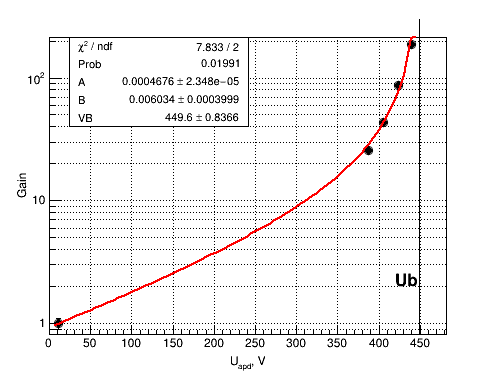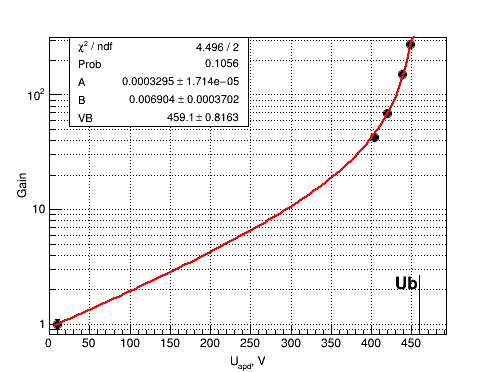# How to draw objects on graph (canvas) according to its size?

I want to draw a vertical line on my graph. But this:

``````mgr->GetXaxis()->SetTitle("U_{apd}, V");
mgr->GetXaxis()->CenterTitle();
mgr->GetXaxis()->SetTitleOffset(1.4);
mgr->GetYaxis()->SetTitle("Gain");
mgr->GetYaxis()->CenterTitle();
mgr->Draw("AP");
//Draw vertical line with text at Ub
TLine* l = new TLine(fitpar, 0, fitpar, 300);
l->Draw("same");
TText* ub = new TText();
ub->DrawText(fitpar-30., 2, "Ub");

c->SetLogy();
c->SetGrid();
``````

provides not very beautiful picture (see Fig.) So how do I know the canvas or graph (which it should be) y-range?GetX1(), GetX2(), GetY1(),GetY2() will return you the pad coordinates

I tried this but do not able to understand the result (see Fig.).

``````mgr->GetXaxis()->SetTitle("U_{apd}, V");
mgr->GetXaxis()->CenterTitle();
mgr->GetXaxis()->SetTitleOffset(1.4);
mgr->GetYaxis()->SetTitle("Gain");
mgr->GetYaxis()->CenterTitle();
mgr->Draw("AP");

c->SetLogy();
c->SetGrid();

//Draw vertical line with text at Ub
TLine* l = new TLine(fitpar, 0, fitpar, c->GetY2());
l->Draw("same");
TText* ub = new TText();
ub->DrawText(fitpar-30., 2, "Ub");
``````How to interpret the return value of `GetY2()`?

Try:```TLine *l = new TLine(fitpar, TMath::Power(10., gPad->GetFrame()->GetY1()), fitpar, TMath::Power(10., gPad->GetFrame()->GetY2()));```

Try this:

``````{
TCanvas *c  = new TCanvas("c","c",0,0,500,500);

const Int_t n = 20;
Double_t x[n], y[n];
for (Int_t i=0;i<n;i++) {
x[i] = 1+i*0.1;
y[i] = 10*sin(x[i]+0.2);
}
gr = new TGraph(n,x,y);
gr->Draw("ACP");

double yl1 = c->GetFrame()->GetY1();
double yl2 = c->GetFrame()->GetY2();
TLine l(1.,yl1,1.,yl2);
l.Draw();

}
``````

It seems it works only if `l` is a pointer to `TLine` but not the `TLine` instance.

Yes if your macro is named you should use a pointer.

OK.
So Do you have an idea why in my initial macro it works different?

See where you call “c->SetLogy();”.

You are right. `SetLogy()` is the thing. But how to deal with this if I want to save the file in `.png` format:

``````gSystem->ProcessEvents();
TImage* img = TImage::Create();
img->WriteImage(figName);
delete img;
delete l;
delete ub;
c->Clear();

``````

If I try to set log-scale before the above piece of code the line becomes shorter.

Sorry, guys. I did not mention that this part of code are in the loop. So I should turn off log-scale after each iteration before the next. How to do this? I tried `SetLogy(kFALSE)` but it does not seem to work.

Can you send a short running reproducer of your problem ?
You only sent pieces of code … that’s hard to debug… Send something with the full logic. Not necessarily the original code (which might be too long) but a small macro rerproducing the effect. Thanks

OK. In a few minutes.

If you are sure that “log Y” is always effective when you create the “TLine” then use the trick from my first post here.

I solved this problem. But not sure in the best way. Here is code:

``````void test(){
TGraph* mgr = new TGraph(3);
mgr->SetMarkerStyle(20);

for (Int_t i = 0; i < 3; i++){
TCanvas* c = new TCanvas("c", "c", 500, 300);
mgr->SetPoint(i, i+1, i+2);

mgr->GetXaxis()->SetTitle("U_{apd}, V");
mgr->GetXaxis()->CenterTitle();
mgr->GetXaxis()->SetTitleOffset(1.4);
mgr->GetYaxis()->SetTitle("Gain");
mgr->GetYaxis()->CenterTitle();
mgr->Draw("AP");

//Draw vertical line with text at Ub
TLine* l = new TLine(2., c->GetFrame()->GetY1(),
2., c->GetFrame()->GetY2());
l->Draw();
TText* ub = new TText();
ub->DrawText(2, 3, "Ub");

gSystem->ProcessEvents();
TImage* img = TImage::Create();
img->WriteImage("test.png");
delete img;
delete l;
delete ub;
delete c;
}
}

``````

You see that every iteration I have to create and delete pointer to canvas.

Yes it can be simpler:

``````void test(){
TGraph* mgr = new TGraph(3);
mgr->SetMarkerStyle(20);
TCanvas* c = new TCanvas("c", "c", 500, 300);
TLine* l = new TLine();
TText* ub = new TText();

for (Int_t i = 0; i < 3; i++) {
mgr->SetPoint(i, i+1, i+2);
mgr->GetXaxis()->SetTitle("U_{apd}, V");
mgr->GetXaxis()->CenterTitle();
mgr->GetXaxis()->SetTitleOffset(1.4);
mgr->GetYaxis()->SetTitle("Gain");
mgr->GetYaxis()->CenterTitle();
mgr->Draw("AP");

//Draw vertical line with text at Ub
l->DrawLine(2., c->GetFrame()->GetY1(),
2., c->GetFrame()->GetY2());
ub->DrawText(2, 3, "Ub");